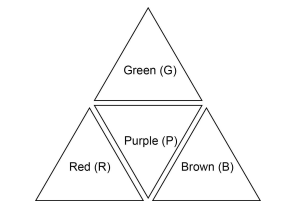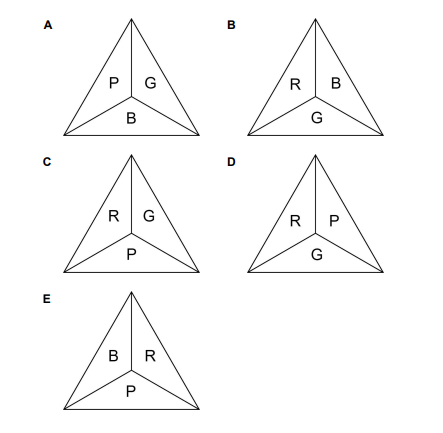# IMAT 2018 Q9 [Tetrahedron View]

A regular tetrahedron is made by fastening together four triangles made from card coloured on one side only, as shown in the diagram below.Which one of the following is NOT a possible view of this tetrahedron when looked at from above?Simple steps to solve word problems:

• Underline key information
• Determine what they are trying to ask, and what you will need to solve it
• Eliminate any non-essential information
• Draw a picture, graph, or equation
• In moments of high stress like exam taking, always work with the paper they give you to avoid careless mistakes.
• Solve.

These questions are very difficult to solve but there is a trick we can use to solve this without having to actually cut out a prism.

Take a look at all of the answers. We want to find two answers that have the same colours (letters) and then compare them. By doing this, we can immediately make this a 50/50, and then we only have to work through 2 patterns instead of all 5. Why can we do this? If we have options with the same letters, they cannot both be correct because it either means that they are superimposable (and therefore they are the same answer), or it means that one is wrong and the other is right. Look and C and D. They both contain R, G, and P. Let’s start by getting the same base, so rotate D in order to have G on the bottom. Now we can see that the difference between C and D is that the position of R and G are switched. But which is the right orientation and which one is the wrong orientation (we are solving for what is NOT a possible view)?

When we can see purple (P) (in the correct image from the question), there has to be red (R) on the left and green (G) on the right when folded up. It may be difficult to see, but fold up the brown (B) side down and the green and red inwards so that the side we cannot see is brown. Draw it beside the image from the question. You will see that this folded-up net matches C. From this, we know that D must be the correct answer because it has R to the right of P and G to the left (which is not what we would see in the original).

Final note: We are using the brown corner as the frame of reference when we fold up because it will be invisible/hidden to us. We know it must contact all sides so this is going help us get the orientation of the sides.

\fcolorbox{red}{grey!30}{Therefore D is the correct answer.}